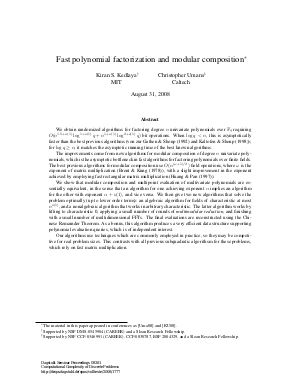Document# Fast polynomial factorization and modular composition

### Authors Kiran Kedlaya, Christopher Umans## File

DagSemProc.08381.5.pdf
• Filesize: 339 kB
• 33 pages

## Cite As

Kiran Kedlaya and Christopher Umans. Fast polynomial factorization and modular composition. In Computational Complexity of Discrete Problems. Dagstuhl Seminar Proceedings, Volume 8381, pp. 1-33, Schloss Dagstuhl - Leibniz-Zentrum für Informatik (2008)
https://doi.org/10.4230/DagSemProc.08381.5

## Abstract

We obtain randomized algorithms for factoring degree \$n\$ univariate polynomials over \$F_q\$ requiring \$O(n^{1.5 + o(1)} log^{1+o(1)} q+ n^{1 + o(1)}log^{2+o(1)} q)\$ bit operations. When \$log q < n\$, this is asymptotically faster than the best previous algorithms (von zur Gathen & Shoup (1992) and Kaltofen & Shoup (1998)); for \$log q ge n\$, it matches the asymptotic running time of the best known algorithms. The improvements come from new algorithms for modular composition of degree \$n\$ univariate polynomials, which is the asymptotic bottleneck in fast algorithms for factoring polynomials over finite fields. The best previous algorithms for modular composition use \$O(n^{(omega + 1)/2})\$ field operations, where \$omega\$ is the exponent of matrix multiplication (Brent & Kung (1978)), with a slight improvement in the exponent achieved by employing fast rectangular matrix multiplication (Huang & Pan (1997)). We show that modular composition and multipoint evaluation of multivariate polynomials are essentially equivalent, in the sense that an algorithm for one achieving exponent \$alpha\$ implies an algorithm for the other with exponent \$alpha + o(1)\$, and vice versa. We then give two new algorithms that solve the problem optimally (up to lower order terms): an algebraic algorithm for fields of characteristic at most \$n^{o(1)}\$, and a nonalgebraic algorithm that works in arbitrary characteristic. The latter algorithm works by lifting to characteristic 0, applying a small number of rounds of {em multimodular reduction}, and finishing with a small number of multidimensional FFTs. The final evaluations are reconstructed using the Chinese Remainder Theorem. As a bonus, this algorithm produces a very efficient data structure supporting polynomial evaluation queries, which is of independent interest. Our algorithms use techniques which are commonly employed in practice, so they may be competitive for real problem sizes. This contrasts with all previous subquadratic algorithsm for these problems, which rely on fast matrix multiplication. This is joint work with Kiran Kedlaya.
##### Keywords
• Modular composition; polynomial factorization; multipoint evaluation; Chinese Remaindering

## Metrics

• Access Statistics
• Total Accesses (updated on a weekly basis)
0